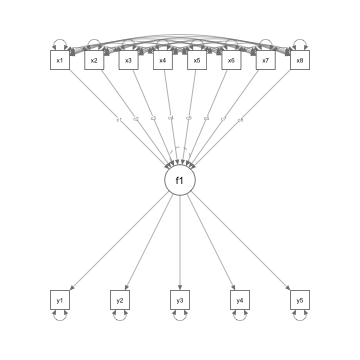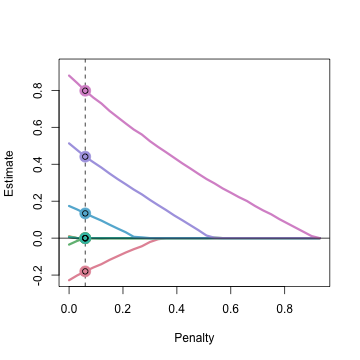``````# install.packages("regsem") # lavaan is a dependency
# install.packages("semPlot")
library(semPlot) # for plotting the model
library(lavaan)
library(regsem)
``````

### Simulate Data

To test out the regsem package, we will first simulate data

``````sim.mod <- "
f1 =~ 1*y1 + 1*y2 + 1*y3+ 1*y4 + 1*y5
f1 ~ 0*x1 + 0*x2 + 0*x3 + 0*x4 + 0*x5 + 0.2*x6 + 0.5*x7 + 0.8*x8
f1~~1*f1"
dat.sim = simulateData(sim.mod,sample.nobs=60,seed=12)
``````

### Run the Model with Lavaan

And then run the model with lavaan so we can better see the structure.

``````run.mod <- "
f1 =~ NA*y1 + y2 + y3+ y4 + y5
f1 ~ c1*x1 + c2*x2 + c3*x3 + c4*x4 + c5*x5 + c6*x6 + c7*x7 + c8*x8
f1~~1*f1
"
lav.out <- sem(run.mod,dat.sim,fixed.x=FALSE)
#summary(lav.out)
parameterestimates(lav.out)[6:13,] # just look at regressions
``````
``````##    lhs op rhs label    est    se      z pvalue ci.lower ci.upper
## 6   f1  ~  x1    c1 -0.228 0.161 -1.412  0.158   -0.543    0.088
## 7   f1  ~  x2    c2  0.010 0.168  0.061  0.951   -0.318    0.339
## 8   f1  ~  x3    c3  0.007 0.150  0.046  0.963   -0.287    0.301
## 9   f1  ~  x4    c4 -0.035 0.150 -0.234  0.815   -0.328    0.258
## 10  f1  ~  x5    c5  0.006 0.174  0.035  0.972   -0.334    0.346
## 11  f1  ~  x6    c6  0.174 0.158  1.103  0.270   -0.136    0.484
## 12  f1  ~  x7    c7  0.513 0.175  2.931  0.003    0.170    0.856
## 13  f1  ~  x8    c8  0.880 0.193  4.558  0.000    0.502    1.259
``````

### Plot the Model

``````semPaths(lav.out)
``````One of the difficult pieces in using the cv_regsem function is that the penalty has to be calibrated for each particular problem. In running this code, I first tested the default, but this was too small given that there were some parameters > 0.4. After plotting this initial run, I saw that some parameters weren't penalized enough, therefore, I increased the penalty jump to 0.03 and with 50 different values this tested a model (at a high penalty) that had all estimates as zero. In some cases it isn't necessary to test a sequence of penalties that would set “large” parameters to zero, as either the model could fail to converge then, or there is not uncertainty about those parameters inclusion.

``````reg.out <- cv_regsem(lav.out,n.lambda=50,type="lasso",jump=0.03,
pars_pen=c("c1","c2","c3","c4","c5","c6","c7","c8"))
``````
``````## Warning in regsem(model = model, lambda = SHRINK, type = type, data
## = data, : WARNING: Model did not converge! It is recommended to try
## multi_optim()
``````
``````## Warning in sqrt(1/diag(V)): NaNs produced
``````
``````## Warning in cov2cor(ImpCov): diag(.) had 0 or NA entries; non-finite result
## is doubtful
``````

In specifying this model, we use the parameter labels to tell cv_regsem which of the parameters to penalize. Equivalently, we could have used the extractMatrices function to identify which parameters to penalize.

``````# not run
extractMatrices(lav.out)["A"]
``````

Additionally, we can specify which parameters are penalized by type: “regressions”, “loadings”, or both c(“regressions”,“loadings”). Note though that this penalizes all parameters of this type. If you desire to penalize a subset of parameters, use either the parameter name or number format for pars_pen.

Next, we can get a summary of the models tested.

``````summary(reg.out)
``````
``````## CV regsem Object
##  Number of parameters regularized: 8
##  Lambda ranging from 0 to 0.93
##  Lowest Fit Lambda: 0.06
##  Metric: BIC
##  Number Converged: 31
``````

## Plot the parameter trajectories

``````plot(reg.out)
``````Here we can see that we used a large enough penalty to set all parameter estimates to zero. However, the best fitting model came early on, when only a couple parameters were zero.

regsem defaults to using the BIC to choose a final model. This shows up in the final_pars object as well as the lines in the plot. This can be changed with the metric argument.

Understand better what went on with the fit

``````head(reg.out\$fits,10)
``````
``````##       lambda conv rmsea      BIC    chisq
##  [1,]   0.00    0     0 2406.615 29.13762
##  [2,]   0.03    0     0 2394.471 29.27645
##  [3,]   0.06    0     0 2390.740 29.63932
##  [4,]   0.09    0     0 2391.229 30.12864
##  [5,]   0.12    0     0 2395.933 30.73821
##  [6,]   0.15    0     0 2392.772 31.67189
##  [7,]   0.18    0     0 2393.806 32.70597
##  [8,]   0.21    0     0 2395.008 33.90813
##  [9,]   0.24    0     0 2396.423 35.32279
## [10,]   0.27    0     0 2397.518 36.41737
``````

One thing to check is the “conv” column. This refers to convergence, with 0 meaning the model converged.

And see what the best fitting parameter estimates are.

``````reg.out\$final_pars[1:13] # don't show variances/covariances
``````
``````## f1 -> y1 f1 -> y2 f1 -> y3 f1 -> y4 f1 -> y5 x1 -> f1 x2 -> f1 x3 -> f1
##    1.008    0.847    0.887    0.960    0.828   -0.180    0.000    0.000
## x4 -> f1 x5 -> f1 x6 -> f1 x7 -> f1 x8 -> f1
##    0.000    0.000    0.134    0.441    0.798
``````

In this final model, we set the regression paths for x2,x3, x4, and x5 to zero. We make a mistake for x1, but we also correctly identify x6, x7, and x8 as true paths .Maximum likelihood estimation with lavaan had p-values > 0.05 for the parameters simulated as zero, but also did not identify the true path of 0.2 as significant (< 0.05).